# MTEL Math: Introduction to Quadratics Chapter Exam

Exam Instructions:

Choose your answers to the questions and click 'Next' to see the next set of questions. You can skip questions if you would like and come back to them later with the yellow "Go To First Skipped Question" button. When you have completed the practice exam, a green submit button will appear. Click it to see your results. Good luck!

Answered 0 of 30

### Page 1

#### Question 4 4. Rewrite the quadratic below in standard form: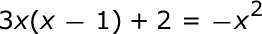### Page 2

#### Question 6 6. Solve this inequality.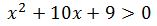### Page 3

#### Question 11 11. Which of the following are the solutions to the equation below?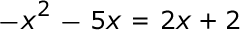#### Question 13 13. What is the b-value of the quadratic equation below?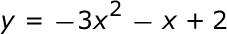#### Question 15 15. Find the roots of the equation.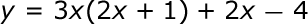### Page 4

#### Question 18 18. Which statement is true about the inequality below?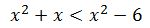#### Question 19 19. Use the quadratic formula to find the solutions to the equation below.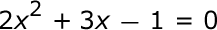### Page 5

#### Question 22 22. What are the zeros in the following quadratic equation?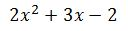#### Question 23 23. Solve the equation.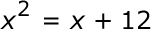### Page 6

#### Question 26 26. Solve the following inequality.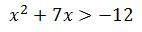#### Question 30 30. Find the roots of the equation below.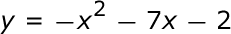#### MTEL Math: Introduction to Quadratics Chapter Exam Instructions

Choose your answers to the questions and click 'Next' to see the next set of questions. You can skip questions if you would like and come back to them later with the yellow "Go To First Skipped Question" button. When you have completed the practice exam, a green submit button will appear. Click it to see your results. Good luck!

Support